### IMO Shortlist 2004 problem A1

Kvaliteta:
Avg: 4,0
Težina:
Avg: 6,0
Let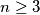$n \geq 3$ be an integer. Let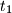$t_1$,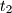$t_2$, ...,$t_n$ be positive real numbers such that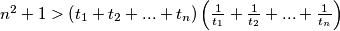$n^2 + 1 > \left( t_1 + t_2 + ... + t_n \right) \left( \frac{1}{t_1} + \frac{1}{t_2} + ... + \frac{1}{t_n} \right)$.

Show that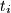$t_i$,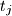$t_j$,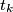$t_k$ are side lengths of a triangle for all$i$,$j$,$k$ with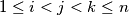$1 \leq i < j < k \leq n$.
Izvor: Međunarodna matematička olimpijada, shortlist 2004